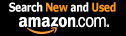CTK Exchange Front Page Movie shortcuts Personal info Awards Reciprocal links Terms of use Privacy Policy Cut The Knot! MSET99 Talk Games & Puzzles Arithmetic/Algebra Geometry Probability Eye Opener Analog Gadgets Inventor's Paradox Did you know?... Proofs Math as Language Things Impossible My Logo Math Poll Other Math sit's Guest book News sit's Recommend this site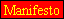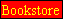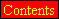|Store|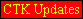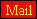CTK Exchange

 Subject: "Spherical integration of any triangular pyramid" Previous Topic | Next Topic
 ConferencesThe CTK ExchangeCollege mathTopic #519Printer-friendly copyEmail this topic to a friend Reading Topic #519
Grantguest
Jul-07-05, 04:09 PM (EST)

"Spherical integration of any triangular pyramid"

 I am trying to develop an algorithm to find the volume of any triangular pyramid by spherical integration using the apex of the pyramid as the origin. I have determined that my integration limits for ro should be: -D/(A*sin(phi)*cos(theta) + B*sin(phi)*sin(theta) + C*cos(phi))if the base is defined by a plane of the form: A*x + B*y + C*z + D = 0However, I am having difficulty constructing the limits of integration of phi (the altitude) as a function of theta (the azimuth).Once I have determined these limits of integration, the limits of integration of theta should be simple.Thanks in advance,Grant

Alert | IP Printer-friendly page | Reply | Reply With Quote | Top
mpdlcguest
Jul-11-05, 10:11 AM (EST)

1. "RE: Spherical integration of any triangular pyramid"
In response to message #0

 Tell me if I am right, just rephrasing your question in this way :Given four points that belong to the surface of an sphere determine the volume of the tetrahedrom forms with these vertexes.It is that your case, as you probably remember the volume of any tetrahedrom is one sixth of the volume of parellelopiped formed with three concurrent edges, which as you also remenber from vector calculus it is the product A*(BxC) . Where symbol * stands for scalar product and x for vector product. And A, B and C means the vector PA, PB and PC naming P the point on the spherical surface taking as origin.Having said that what is now to be done it is to translate the spherical coordinates of the four points into rectangular coordinates. Xa = Rcos(lat)cos(long) ; Ya = Rcos(lat)sin(long) ; Za = Rsin(lat) ;and same for the other points, Now substracting the coordinates of P you will obtain the components of vector A, B, and C A is : (Xa-Xp)i +(Ya-Yp)j +(Za-Zp)k B is : (Xb-Xp)i +(Yb-Yp)j +(Zb-Zp)k C is : (Xc-Xp)i +(Yc-Yp)j +(Zc-Zp)k Where R stands for the sphere radius, lat means latitude and long means longitude or you can use altitude and azimuthso the volume is 1/6 of the determinant Ax Ay Az Bx By Bz Cx Cy Cz Since you want the algorithm related to the spherical coordinates leave indicated in the formula the latitude and longitude of the points.

Alert | IP Printer-friendly page | Reply | Reply With Quote | Top

 Conferences | Forums | Topics | Previous Topic | Next Topic
 Select another forum or conference Lobby The CTK Exchange (Conference)   |--Early math (Public)   |--Middle school (Public)   |--High school (Public)   |--College math (Public)   |--This and that (Public)   |--Guest book (Public) Educational Press (Conference)   |--No Child Left Behind (Public)   |--Math Wars (Public)   |--Mathematics and general education (Public)You may be curious to have a look at the old CTK Exchange archive.
Please do not post there.